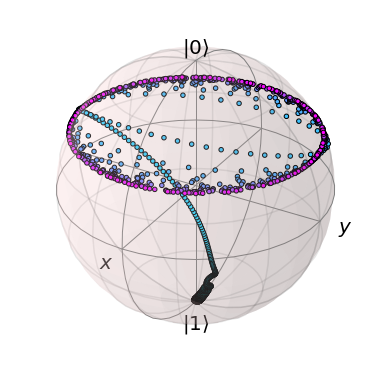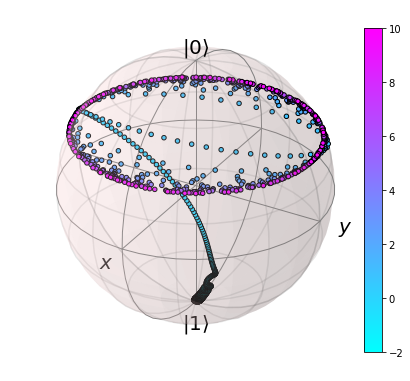# Adding a colorbar to a Bloch Sphere¶

In :
import numpy as np
import matplotlib as mpl
import matplotlib.pyplot as plt
from matplotlib import cm
from mpl_toolkits.mplot3d import Axes3D
from qutip import *
from qutip.ipynbtools import version_table
%matplotlib inline


## Do a closed Landau-Zener Evolution¶

In :
delta = 0.5 * 2 * np.pi
v = 2.0 * 2 * np.pi # sweep rate

H0 = delta/2.0 * sigmax()
H1 = v/2.0 * sigmaz()
H = [H0, [H1, 't']]
psi0 = basis(2,0)

sm = destroy(2)
sx=sigmax();
sy=sigmay()
sz=sigmaz()
expt_ops = [sm.dag() * sm,sx,sy,sz]

tlist = np.linspace(-10.0, 10.0, 1500)
expt_list = sesolve(H, psi0, tlist, expt_ops).expect


## Generate a Bloch Sphere with Multi-Colored Points¶

Note that I need to call b.show here so that I can grab a Figure instance later

In :
b = Bloch()
## normalize colors to times in tlist ##
nrm = mpl.colors.Normalize(-2,10)
colors = cm.cool(nrm(tlist))

## add data points from expectation values ##
expt_list,
-expt_list],'m')

## customize sphere properties ##
b.point_color = list(colors)
b.point_marker = ['o']
b.point_size = 

b.zlpos = [1.1,-1.2]

b.show()## Add New Axis to Bloch Figure¶

In :
left, bottom, width, height = [0.98, 0.05, 0.05, 0.9]
ax2 = b.fig.add_axes([left, bottom, width, height])

mpl.colorbar.ColorbarBase(ax2, cmap=cm.cool,
norm=nrm,
orientation='vertical');


Currently I need to call b.fig as replotting figures in a notebook is a bit tricky. However, this is likely to be improved in the future.

In :
b.fig

Out:In :
version_table()

Out:
SoftwareVersion
QuTiP4.3.0.dev0+4a9c6fd1
Numpy1.13.1
SciPy0.19.1
matplotlib2.0.2
Cython0.26.1
Number of CPUs2
BLAS InfoINTEL MKL
IPython6.1.0
Python3.6.2 |Anaconda, Inc.| (default, Sep 21 2017, 18:29:43) [GCC 4.2.1 Compatible Clang 4.0.1 (tags/RELEASE_401/final)]
OSposix [darwin]
Mon Oct 09 11:24:04 2017 MDT